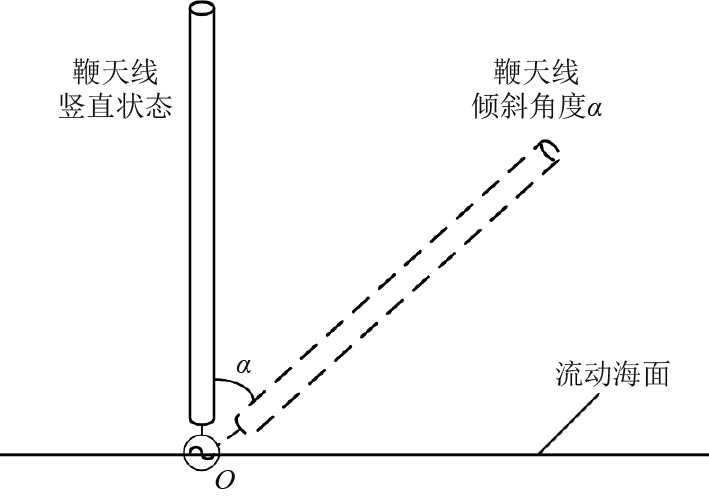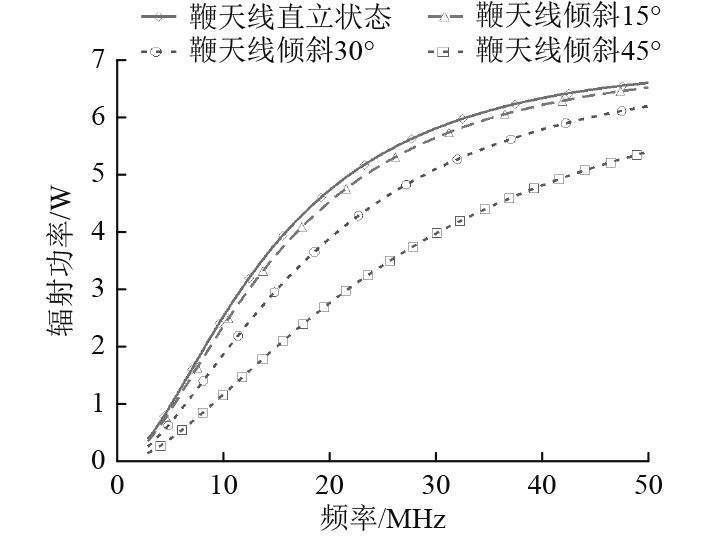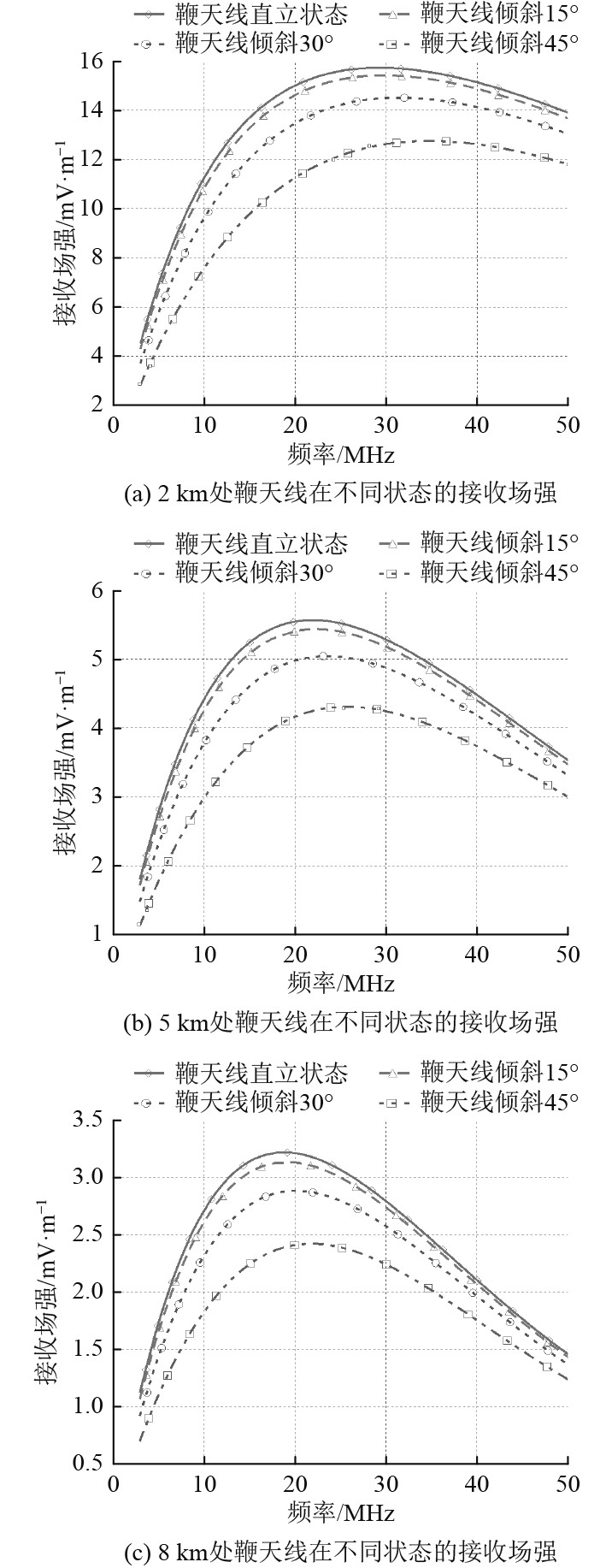﻿ 水面无人艇搭载鞭天线最佳工作频率分析
 舰船科学技术2023, Vol. 45Issue (1): 90-93    DOI: 10.3404/j.issn.1672-7649.2023.01.016PDF

Analysis of the best working frequency of whip antenna on surface unmanned vehicle
XIU Meng-lei, LI Li-hua, HOU Wen-da, WANG Long-fei
Department of Communication Engineering, Naval University of Engineering, Wuhan 430000, China
Abstract: In order to ensure that the surface unmanned vehicle and the receiving target achieve close-range communication, the whip antenna radiation and electromagnetic wave propagation characteristics are combined in the 3～50 MHz frequency band to determine the maximum field strength that can be received at different distances. The radiation efficiency of the whip antenna on the sea surface under multiple conditions is calculated and the simulation results are obtained; the ground wave propagation attenuation factor model is used for quantitative analysis and simulation experiments, and finally the best working frequency of the whip antenna at different distances under various conditions when working on the sea surface is obtained. Theoretical analysis and simulation results show that as the distance increases, the corresponding reception field strength of the whip antenna in various states decreases, with the increase of the tilt angle, the optimal operating frequency shows an increasing trend, and the reception field strength continues to decrease.
Key words: whip antenna radiation     electromagnetic wave propagation     best working frequency     receiving field strength
0 引　言

1 辐射性能分析

 $D(\theta ,\varphi ) = \frac{{S(\theta ,\varphi )}}{{{S_0}}} = \frac{{{{\left| {E(\theta ,\varphi ,r)} \right|}^2}/240{\text{π}} }}{{{P_r}/4{\text{π}} {r^2}}} 。$ (1)

 $\eta=\frac{P_{r}}{P_{i z}}=\frac{r^{2}|E(\theta, \varphi, r)|^{2}}{60 P_{i z} D(\theta, \varphi)} \times 100 {\text{%}} 。$ (2)

 $D(\theta ,\varphi ) = \frac{{4{\text{π}} {F^2}(\theta ,\varphi )}}{{\displaystyle\int_0^{2{\text{π}} } {\displaystyle\int_0^{\text{π}} {{F^2}(\theta ,\varphi ){\rm{d}}\theta {\rm{d}}\varphi } } }}。$ (3)图 1 鞭天线在流动海面工作状态图 Fig. 1 Working state diagram of whip antenna in the flowing sea water surface图 2 1 m鞭天线在3～50 MHz频段倾斜不同角度时的辐射功率对比 Fig. 2 Comparison of radiated power of 1 m whip antenna when tilted at different angles in the 3～50 MHz frequency band

1）对于在海面上工作的鞭天线，在3～50 MHz的频段下，对于1 m鞭天线，随着频率的不断增加，鞭天线辐射功率也不断增加。这是因为在该范围内，天线的辐射电阻会随着 $h/\lambda$ 的增大而增大，在物理尺寸一定的情况下，电尺寸将直接决定辐射功率。

2）天线的倾斜角度越大，天线的辐射功率不断减小，这是因为倾斜角度越大，天线与海面地损耗不断增大，此时海水会吸收更多的能量，导致天线辐射能力有所下降。

2 传播特性分析

 $\left| E \right| = \frac{{173\sqrt {{P_r}D} }}{r}A。$ (4)

 ${d_0} = 80{f^{ - 1/3}}，$ (5)

 $A = \frac{{2 + 0.3d}}{{2 + d + 0.6{d^2}}} - \sqrt {\frac{d}{2}} \exp ( - 1.44d{\rm{log}}{\varepsilon _r}) \cdot {\rm{sin}}\beta 。$ (6)

 $d \approx 1.745 \times {10^{ - 4}} \cdot \frac{{f \cdot \cos \beta }}{\sigma } \cdot \frac{{r \times {{10}^8}}}{\lambda }，$ (7)
 $\beta = - {\tan ^{ - 1}}\frac{{({\varepsilon _r} + 1) \cdot f}}{{1.8 \times {{10}^4}\sigma }} 。$ (8)

3 接收场强分析图 3 鞭天线在3～50 MHz频段不同倾斜角度在部分距离的接收场强 Fig. 3 Receiving field strength of whip antenna in the 3～50 MHz frequency band with different tilt angles at partial distance表 1 3～50 MHz频段1 m鞭天线不同倾斜角度在10 km内的最佳工作频率 Tab.1 The best working frequency of 1 m whip antenna in the 3～50 MHz frequency band with different tilt angles within 10 km
4 结　语

  李雪, 胡海驹, 王伟平, 等. 水面无人艇远距离通信系统设计与实现[J]. 电信科学, 2021, 37(7): 48-56. LI X, HU H X, WANG W P, et al. Design and implementation of long-distance communication system for surface unmanned craft[J]. Telecommunications Science, 2021, 37(7): 48-56.  马银圣, 何绍林, 聂贺峰. 一种短波宽带鞭天线的设计方法[J]. 电波科学学报, 2018, 33(3): 365-370. MA Y S, HE S L, NIE H F. A short-wave broadband whip antenna design method[J]. Chinese Journal of Radio Science, 2018, 33(3): 365-370. DOI:10.13443/j.cjors.2018042301  WANG H F, LIU C. Switchable design of a frequency reconfigurable broadband whip antenna in high frequency[J]. International Journal of Microwave and Wireless Technologies, 2020, 13(5): 454-462.  WANG H F, LIU C. Optimal pattern synthesis of linear array and broadband design of whip antenna using grasshopper optimization algorithm[J]. International Journal of Antennas and Propagation, 2020, 1-14.  刘桐, 王永斌, 李丽华, 等. 海洋环境下小型短波鞭天线调谐性能仿真分析[J]. 计算机仿真, 2018, 35(5): 177-181. LIU T, WANG Y B, LI L H, et al. Simulation analysis of tuning performance of small short-wave whip antenna in marine environment[J]. Computer Simulation, 2018, 35(5): 177-181. DOI:10.3969/j.issn.1006-9348.2018.05.037  王善进, 王亚迅. 无线电波沿光滑海面传播损耗的计算[J]. 微波学报, 2005(6): 27-29. WANG S J, WANG Y X. Calculation of radio wave propagation loss along smooth sea surface[J]. Journal of Microwaves, 2005(6): 27-29. DOI:10.3969/j.issn.1005-6122.2005.06.006  郭建炎, 王剑莹, 龙云亮. 基于抛物方程法的粗糙海面电波传播分析[J]. 通信学报, 2009, 30(6): 47-52. GUO J Y, WANG J Y, LONG Y L. Analysis of Radio Wave Propagation in Rough Sea Based on Parabolic Equation Method[J]. Journal of Communications, 2009, 30(6): 47-52. DOI:10.3321/j.issn:1000-436X.2009.06.008  赵雨薇, 迟迅, 任佳. 基于ITM模型的海上移动信道传输模型[J]. 电子技术应用, 2014, 40(7): 106-108+111. ZHAO Y W, CHI X, REN J. Maritime mobile channel transmission model based on ITM model[J]. Application of Electronic Technology, 2014, 40(7): 106-108+111. DOI:10.3969/j.issn.0258-7998.2014.07.032  许浩, 张儒申, 徐卸土. 3.5GHz频段电波传播特性研究[J]. 电信科学, 2018, 34(S2): 140-145. XU H, ZHANG R S, XU X S. Research on the Propagation Characteristics of Radio Waves in the 3.5GHz Frequency Band[J]. Telecommunications Science, 2018, 34(S2): 140-145.  全厚德, 兰田, 孙慧贤, 等. 多子模型方法预测电波传播损耗研究[J]. 火力与指挥控制, 2018, 43(9): 94-98. QUAN H D, LAN T, SUN H X, et al. Research on prediction of radio wave propagation loss by multi-submodel method[J]. Firepower and Command Control, 2018, 43(9): 94-98. DOI:10.3969/j.issn.1002-0640.2018.09.020